# NCERT Exemplar Class 11 Physics Solutions for Chapter 12 - Thermodynamics

NCERT Exemplar Solutions for Class 11 Physics Chapter 12 Thermodynamics are crucial study materials to understand important topics easily. Thermodynamics Physics Class 11 questions provided here are taken from the NCERT Exemplar book, previous years’ question papers and sample papers.

By studying the NCERT Exemplar Class 11 Physics Chapter 12, you will get the extra knowledge necessary to face the final examination and graduate entrance examinations confidently. This solution provides Class 11 Physics Thermodynamics MCQ questions, CBSE Class 11 Physics Thermodynamics important questions, numerical problems, worksheets and exercises that will guide you to prepare Thermodynamics Physics Class 11 NCERT notes.

The branch of Physics which deals with heat and temperature is called thermodynamics. It has a direct relation to work and energy. Thermodynamics has a wide range of applications in fields such as science and engineering. Some of the major fields of engineering where thermodynamics is applied are chemical engineering, aerospace engineering, mechanical engineering, material science, economics, etc.

## Different Laws of Thermodynamics

1. Zeroth law of thermodynamics
2. First law of thermodynamics
3. Second law of thermodynamics
4. Third law of thermodynamics

Check out the NCERT Exemplar Class 11 Physics Chapter 12 Thermodynamics PDF below.

## Download the PDF of NCERT Exemplar Class 11 Physics Chapter 12 Thermodynamics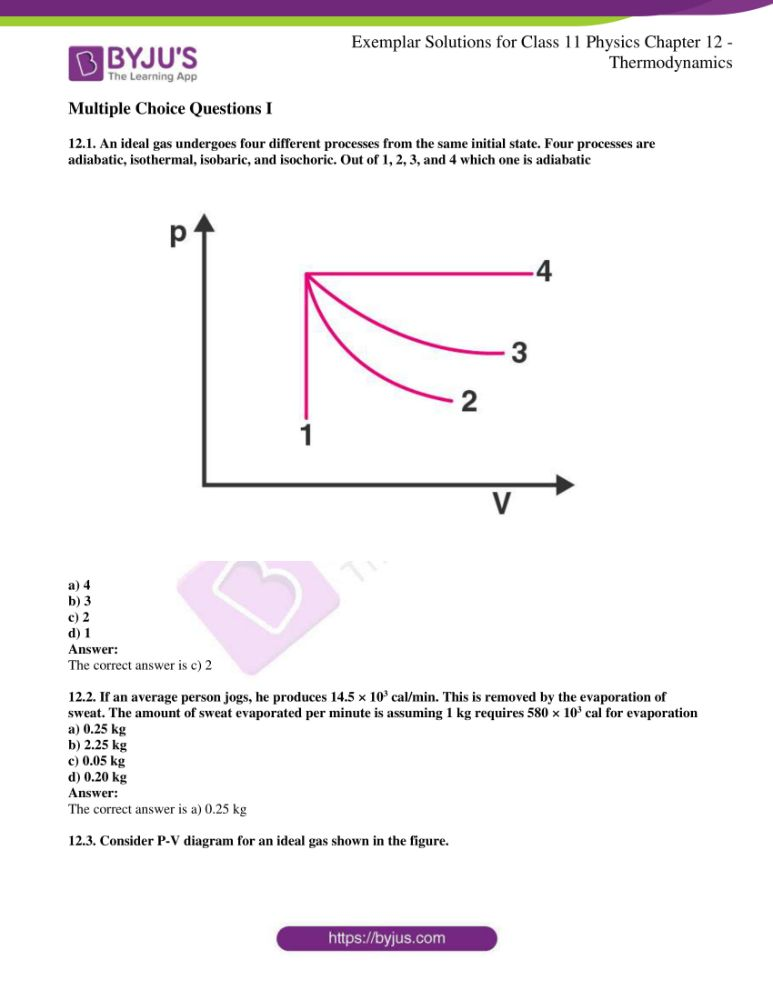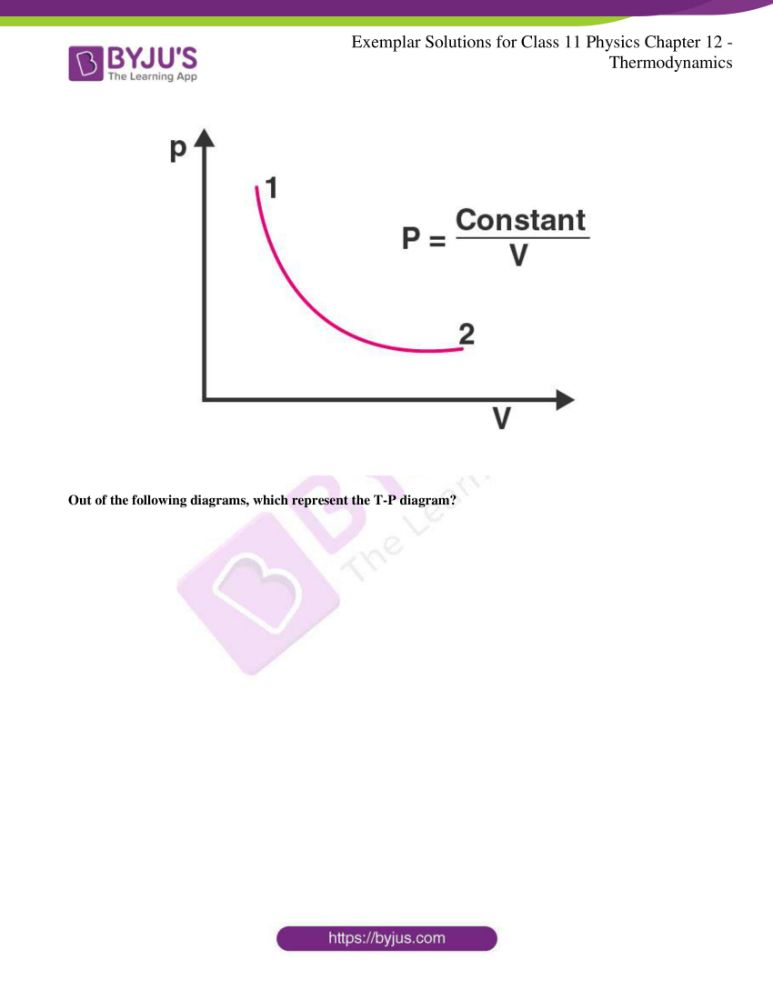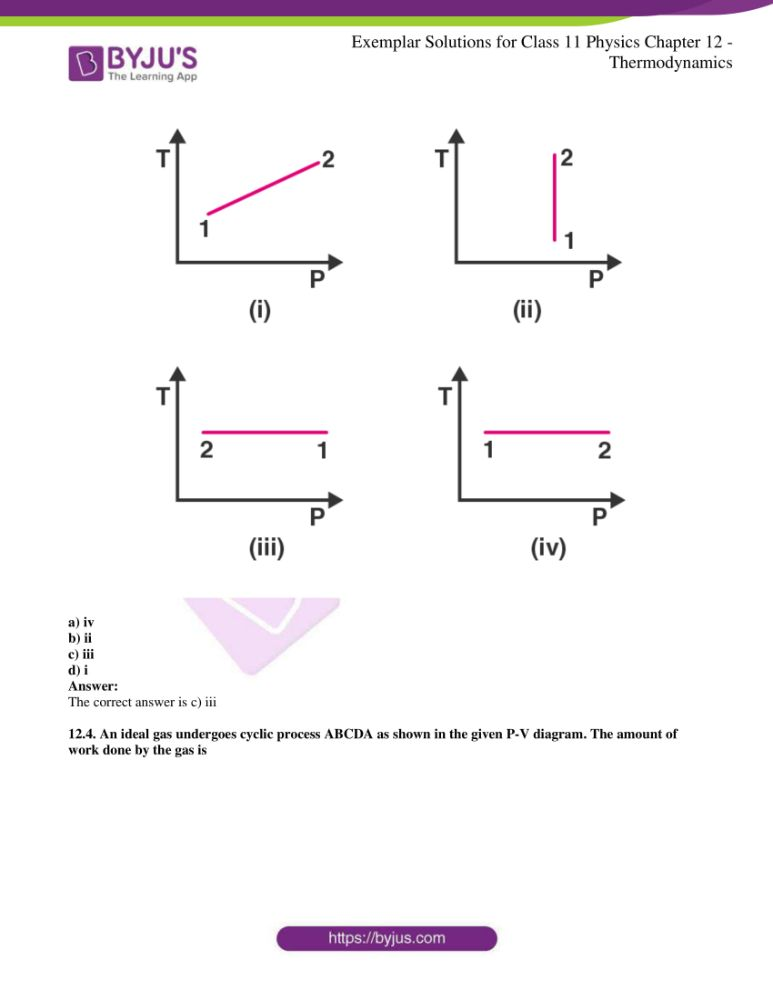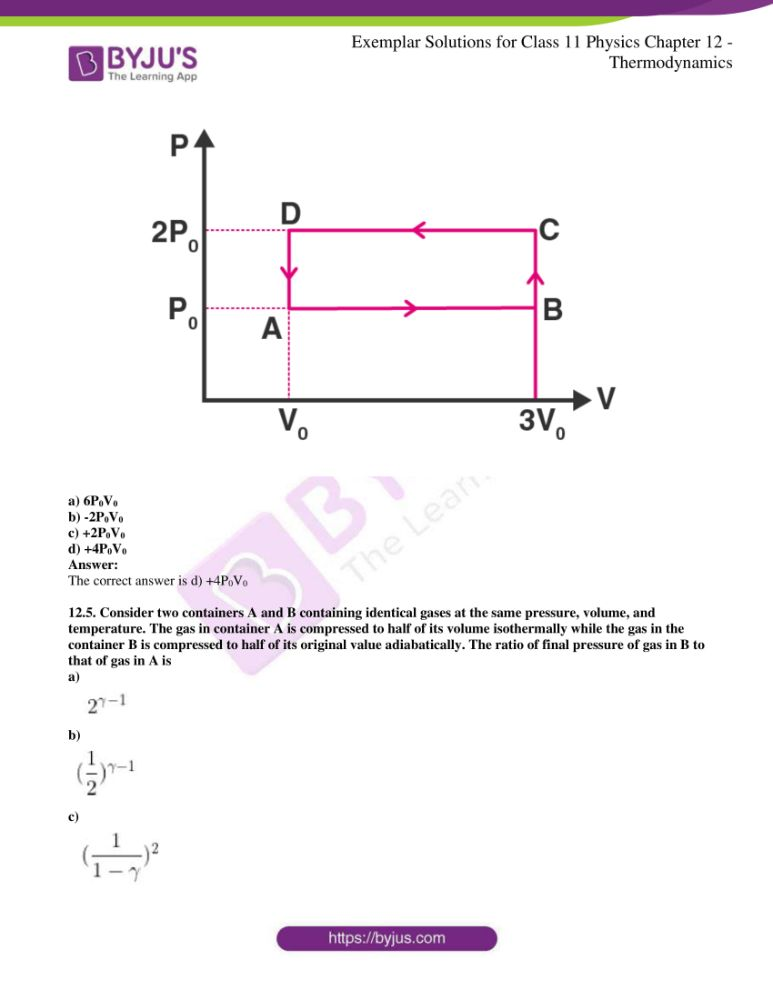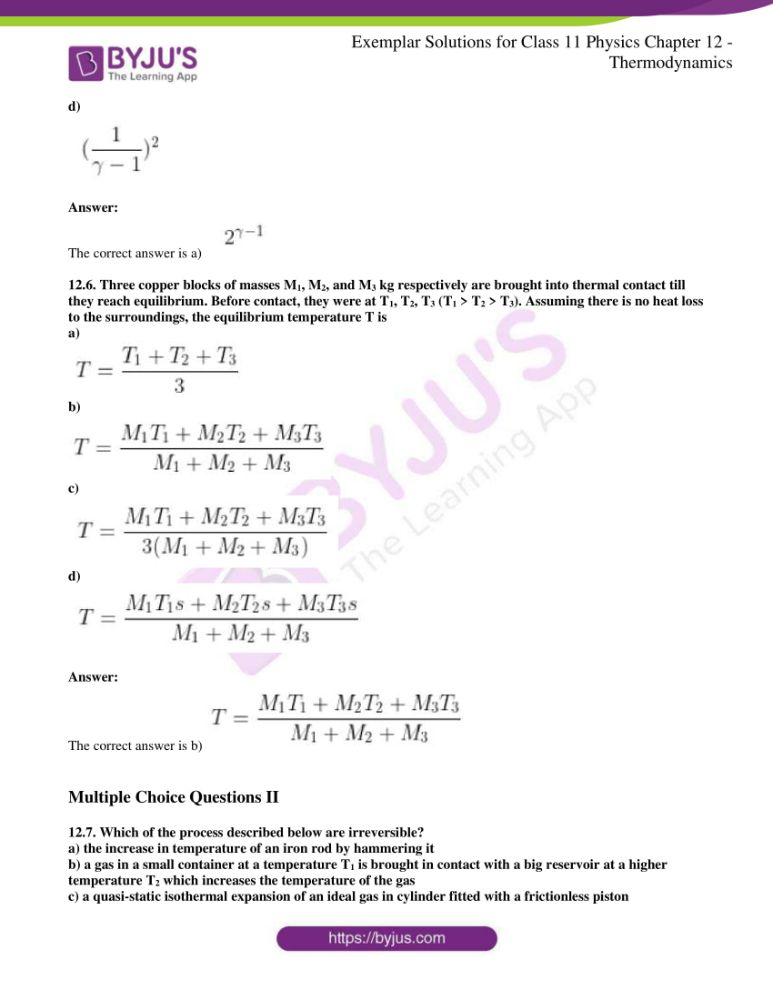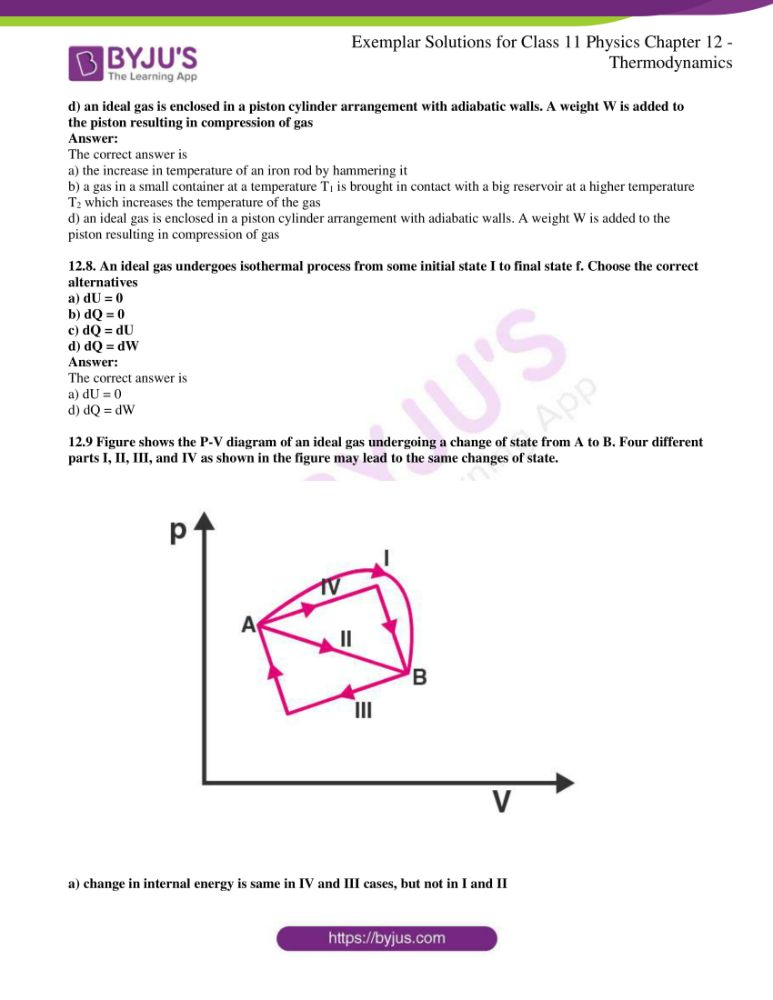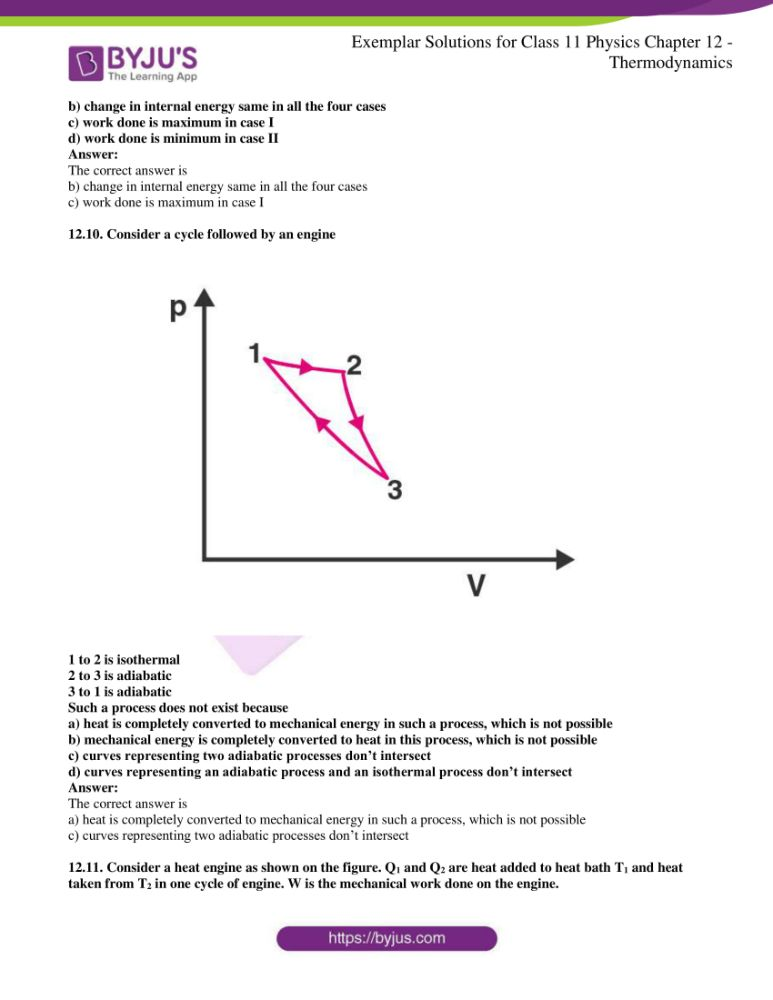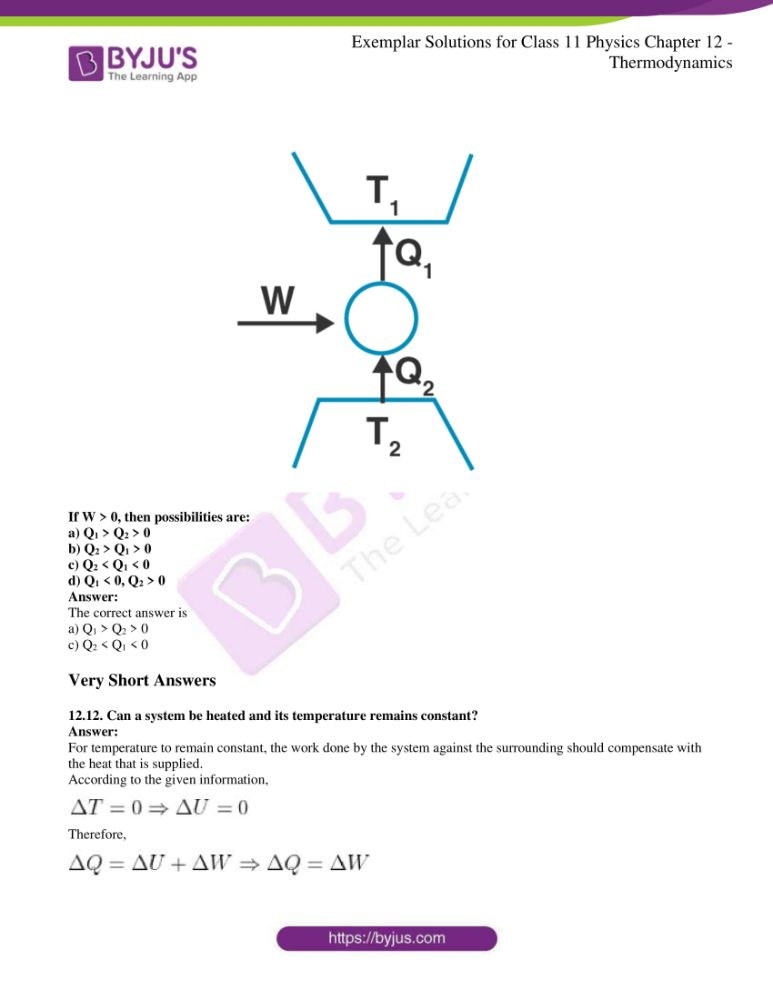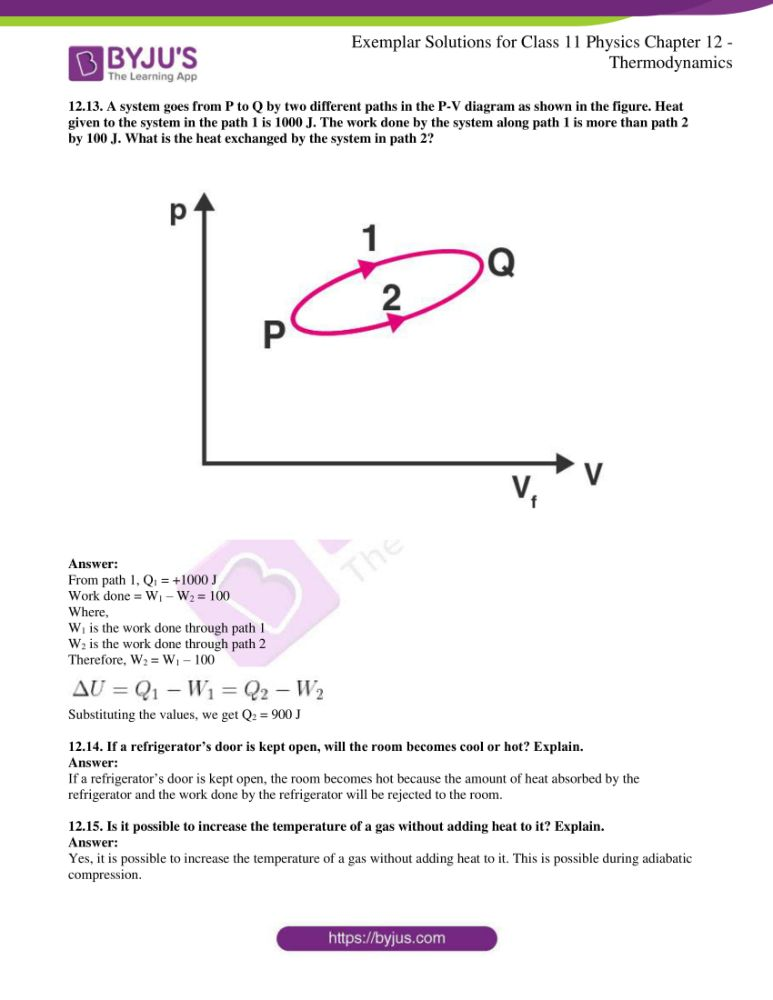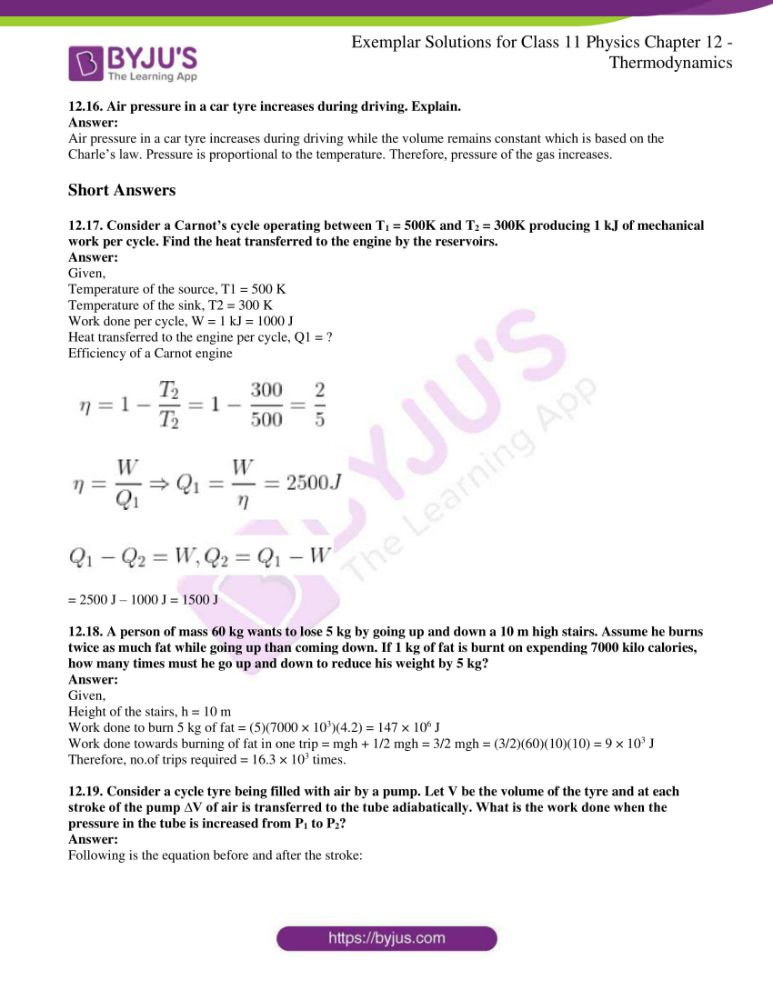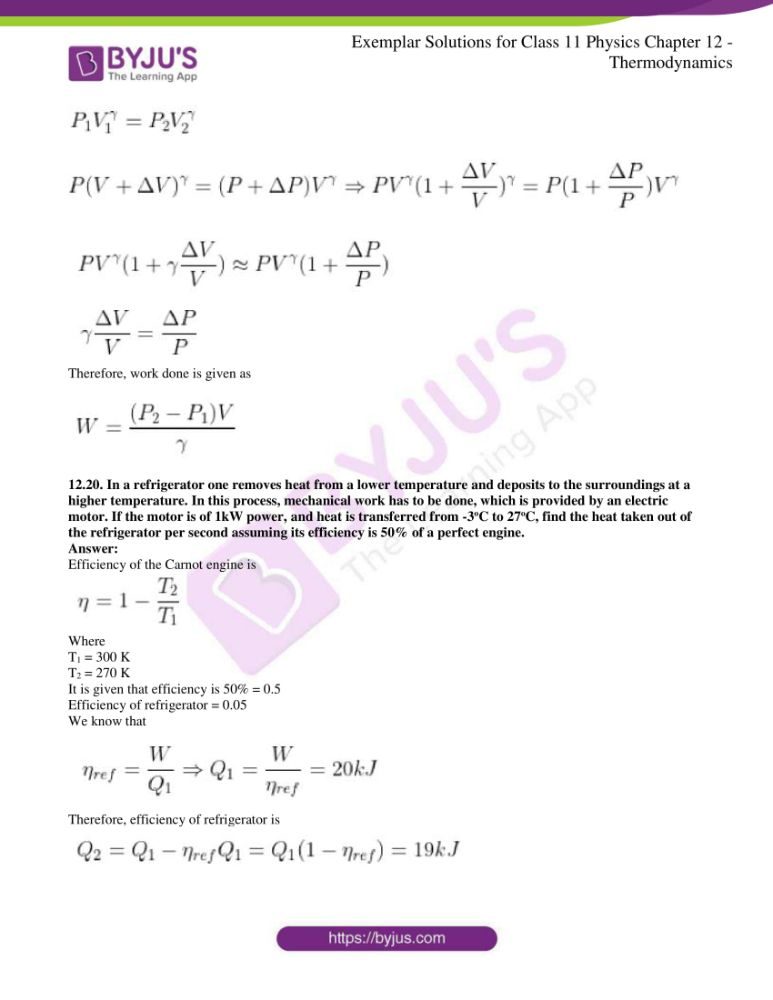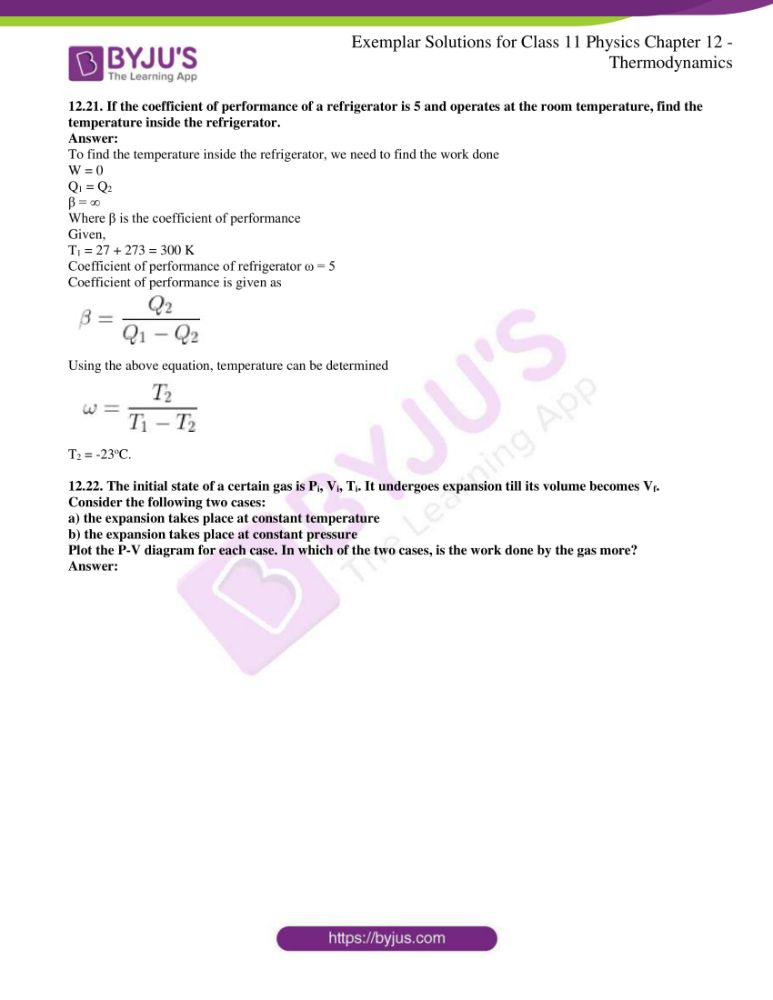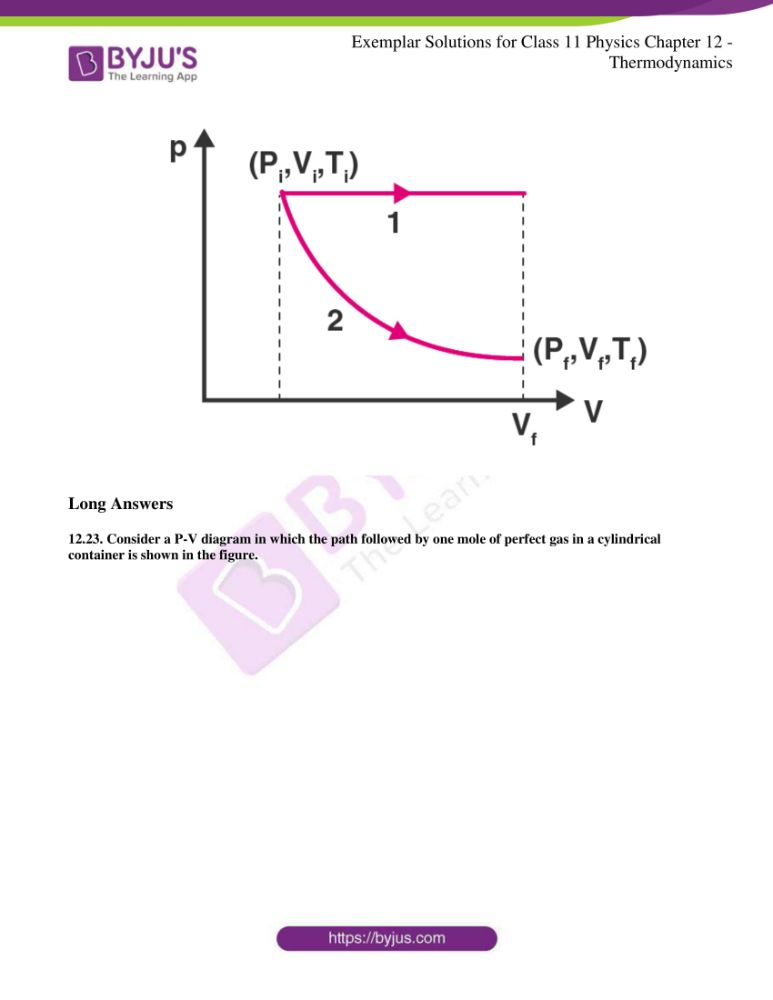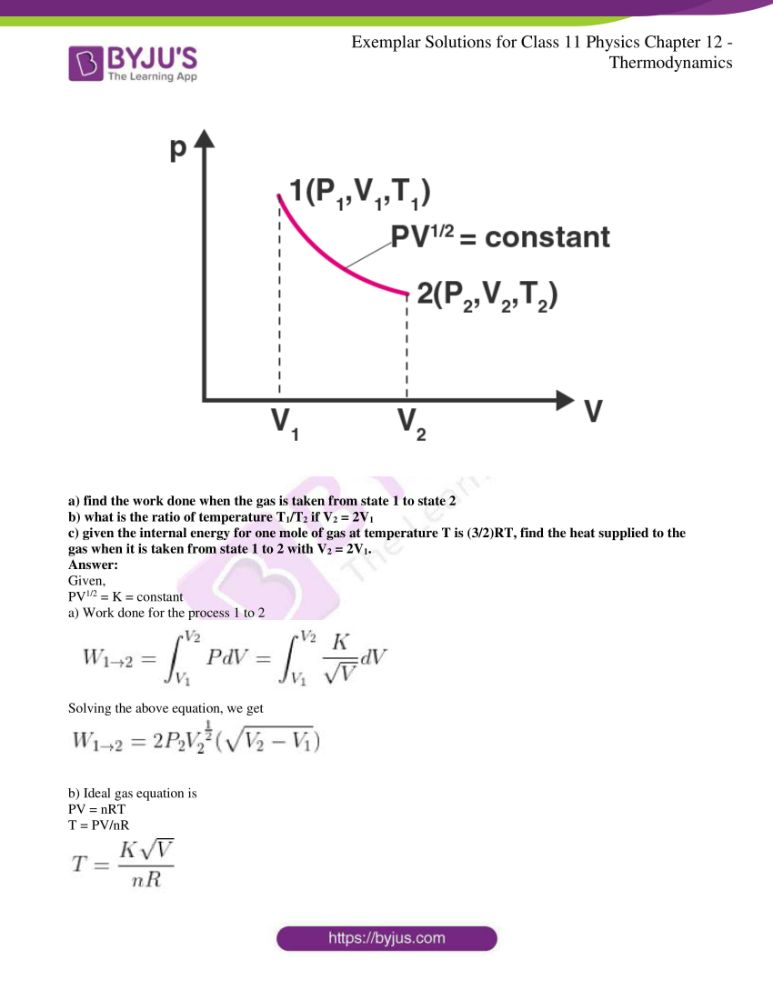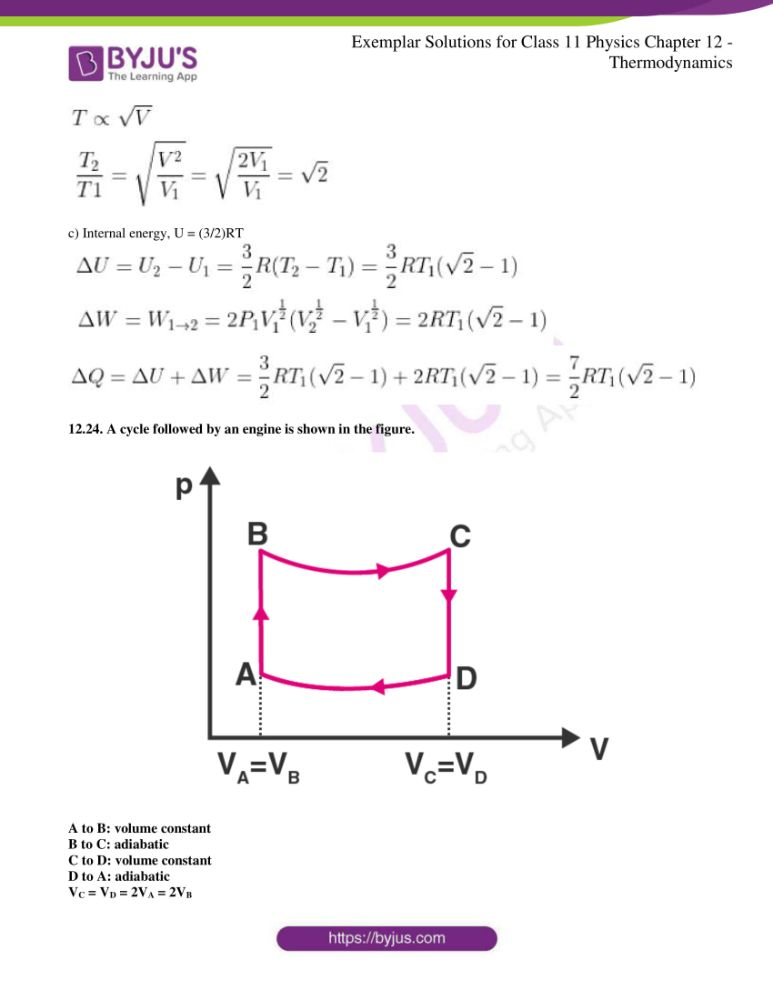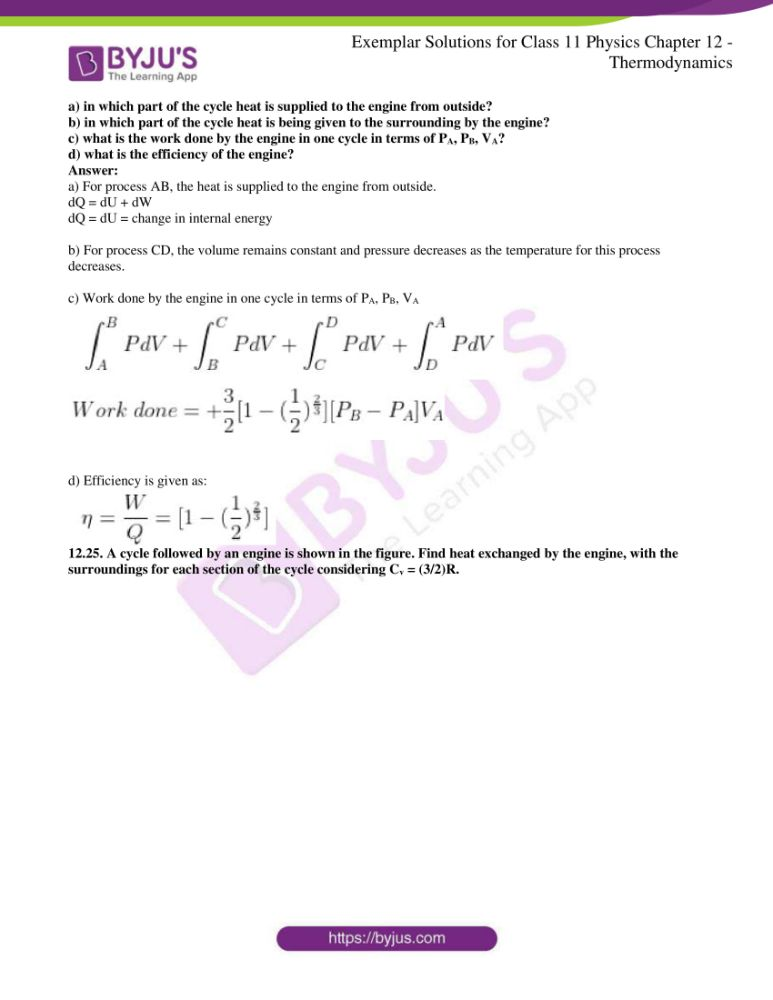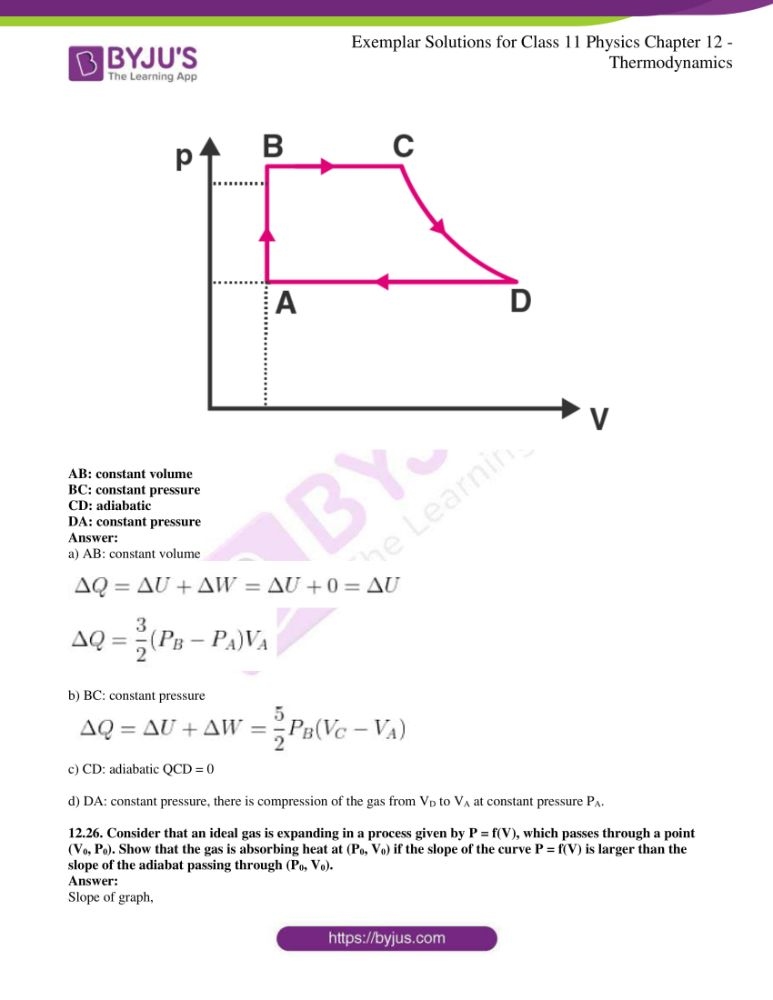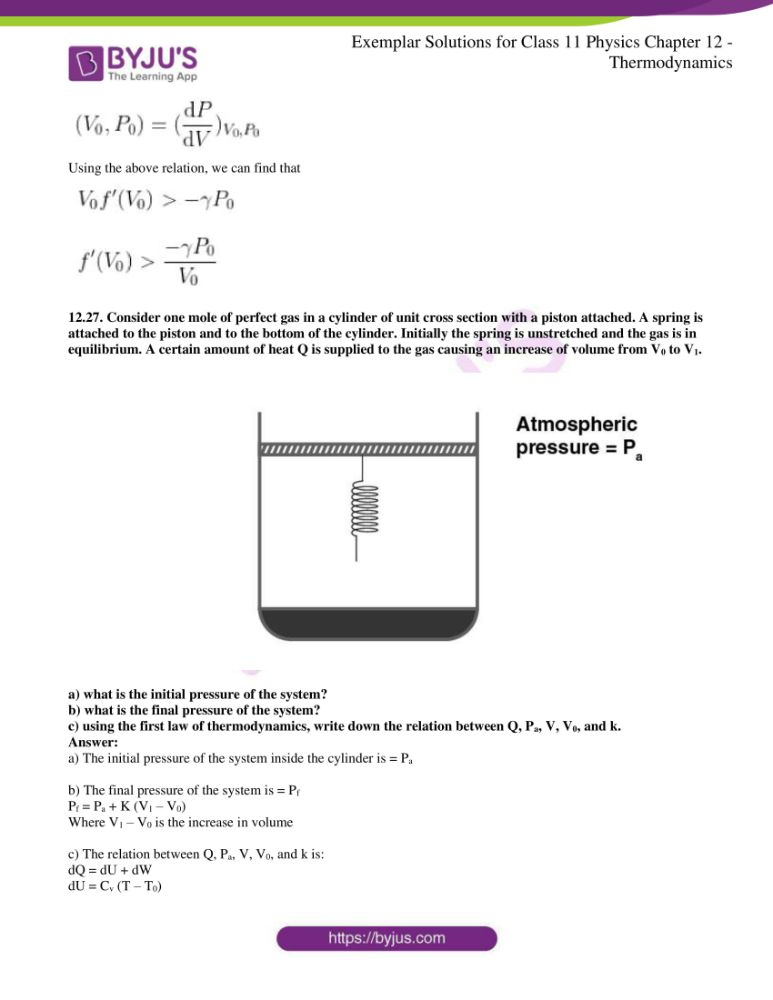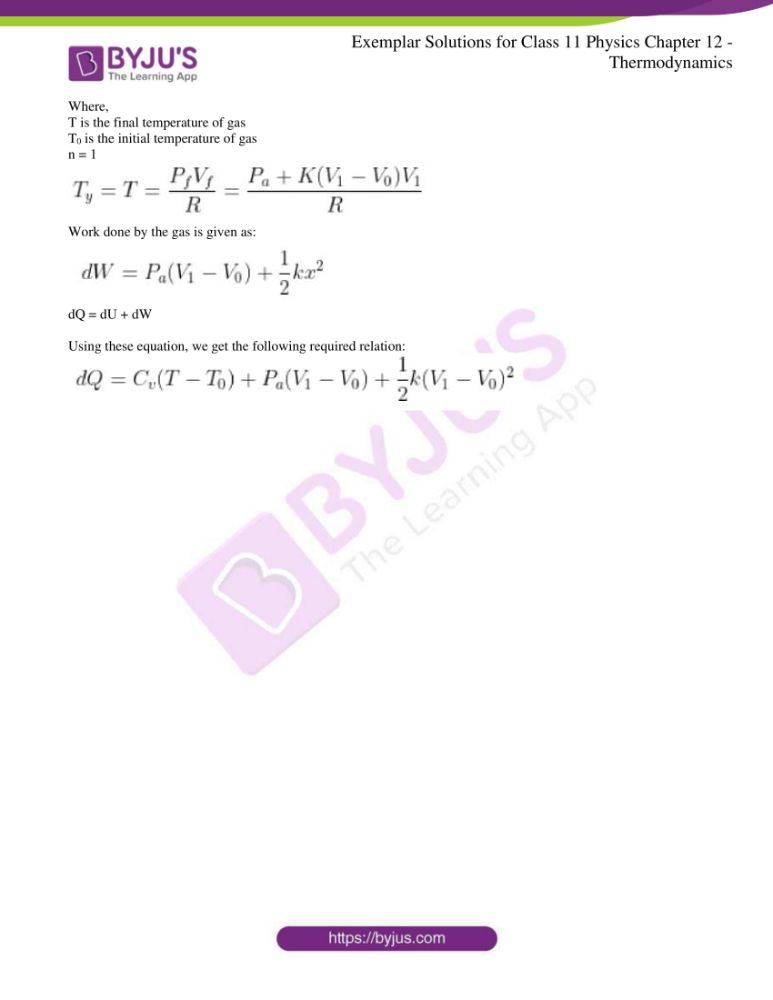### Multiple Choice Questions I

12.1. An ideal gas undergoes four different processes from the same initial state. Four processes are adiabatic, isothermal, isobaric, and isochoric. Out of 1, 2, 3, and 4 which one is adiabatic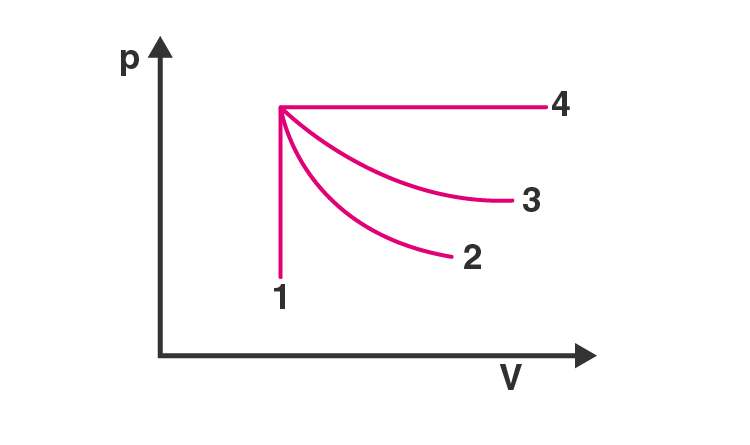a) 4

b) 3

c) 2

d) 1

The correct answer is c) 2

12.2. If an average person jogs, he produces 14.5 × 103 cal/min. This is removed by the evaporation of sweat. The amount of sweat evaporated per minute is assuming 1 kg requires 580 × 103 cal for evaporation

a) 0.25 kg

b) 2.25 kg

c) 0.05 kg

d) 0.20 kg

The correct answer is a) 0.25 kg

12.3. Consider P-V diagram for an ideal gas shown in the figure.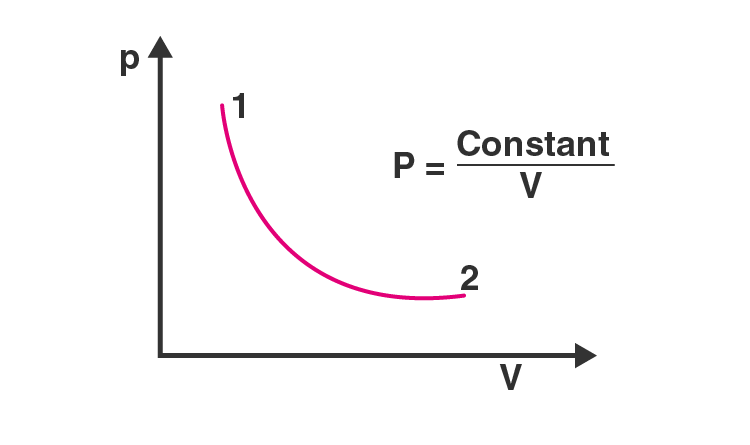Out of the following diagrams, which represent the T-P diagram?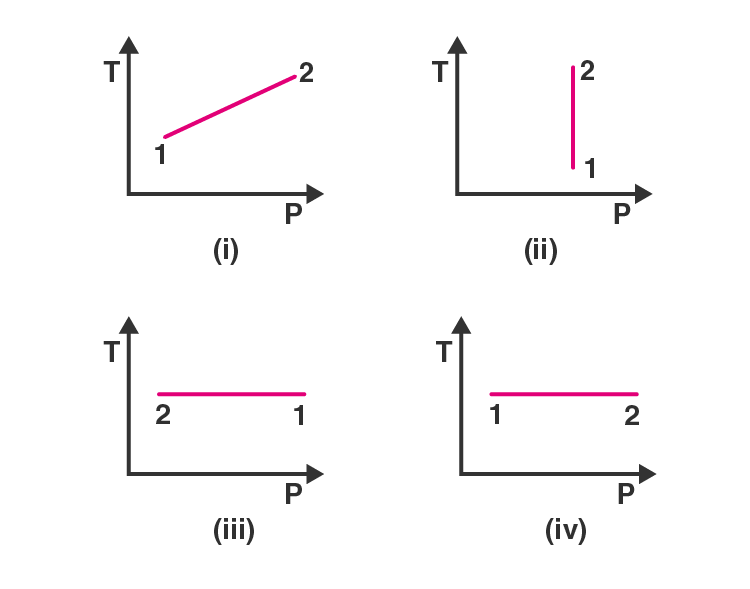a) iv

b) ii

c) iii

d) i

The correct answer is c) iii

12.4. An ideal gas undergoes cyclic process ABCDA as shown in the given P-V diagram. The amount of work done by the gas is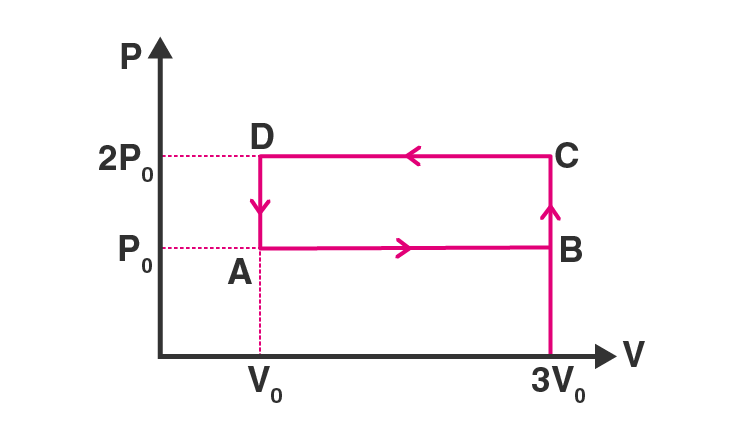a) 6P0V0

b) -2P0V0

c) +2P0V0

d) +4P0V0

The correct answer is d) +4P0V0

12.5. Consider two containers A and B containing identical gases at the same pressure, volume, and temperature. The gas in container A is compressed to half of its volume isothermally while the gas in the container B is compressed to half of its original value adiabatically. The ratio of final pressure of a gas in B to that of gas in A is

a)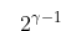b)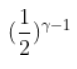c)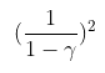d)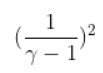12.6. Three copper blocks of masses M1, M2, and M3 kg respectively are brought into thermal contact till they reach equilibrium. Before contact, they were at T1, T2, T3 (T1 > T2 > T3). Assuming there is no heat loss to the surroundings, the equilibrium temperature T is

a)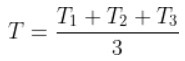b)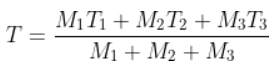c)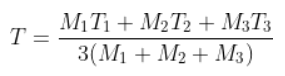d)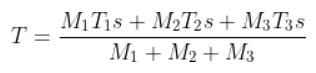### Multiple Choice Questions II

12.7. Which of the process described below are irreversible?

a) the increase in temperature of an iron rod by hammering it

b) a gas in a small container at a temperature T1 is brought in contact with a big reservoir at a higher temperature T2 which increases the temperature of the gas

c) a quasi-static isothermal expansion of an ideal gas in a cylinder fitted with a frictionless piston

d) an ideal gas is enclosed in a piston-cylinder arrangement with adiabatic walls. A weight W is added to the piston resulting in compression of gas

a) the increase in temperature of an iron rod by hammering it

b) a gas in a small container at a temperature T1 is brought in contact with a big reservoir at a higher temperature T2 which increases the temperature of the gas

d) an ideal gas is enclosed in a piston-cylinder arrangement with adiabatic walls. A weight W is added to the piston resulting in compression of gas

12.8. An ideal gas undergoes isothermal process from some initial state I to final state f. Choose the correct alternatives

a) dU = 0

b) dQ = 0

c) dQ = dU

d) dQ = dW

a) dU = 0

d) dQ = dW

12.9 Figure shows the P-V diagram of an ideal gas undergoing a change of state from A to B. Four different parts I, II, III, and IV as shown in the figure may lead to the same changes of state.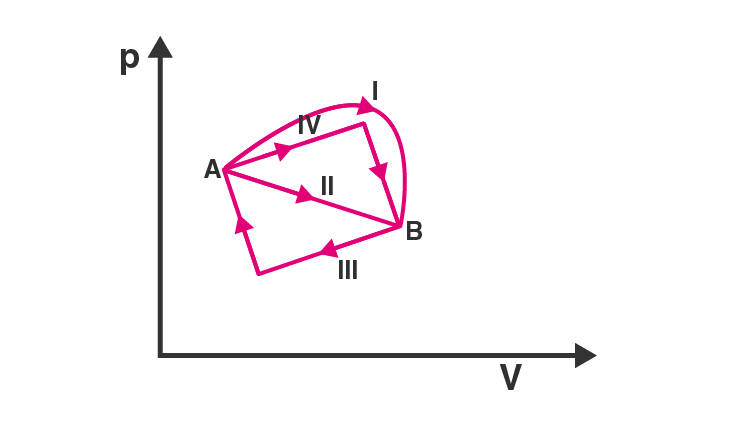a) change in internal energy is the same in IV and III cases, but not in I and II

b) change in internal energy same in all the four cases

c) work done is maximum in case I

d) work done is minimum in case II

b) change in internal energy same in all the four cases

c) work done is maximum in case I

12.10. Consider a cycle followed by an engine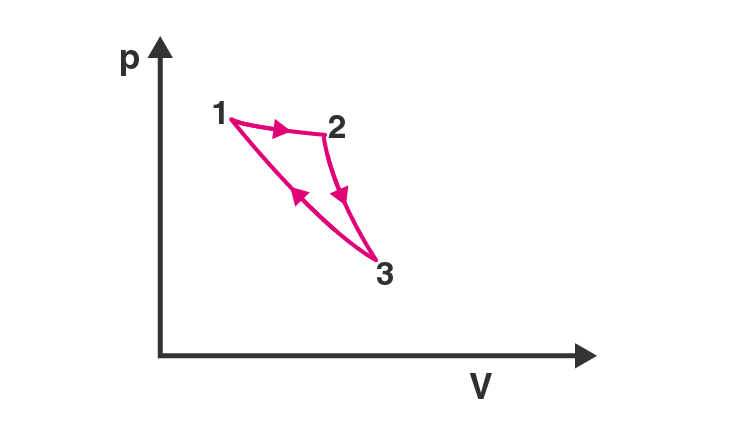1 to 2 is isothermal

Such a process does not exist because

a) heat is completely converted to mechanical energy in such a process, which is not possible

b) mechanical energy is completely converted to heat in this process, which is not possible

c) curves representing two adiabatic processes don’t intersect

d) curves representing an adiabatic process and an isothermal process don’t intersect

a) heat is completely converted to mechanical energy in such a process, which is not possible

c) curves representing two adiabatic processes don’t intersect

12.11. Consider a heat engine as shown on the figure. Q1 and Q2 are heat added to heat bath T1 and heat taken from T2 in one cycle of the engine. W is the mechanical work done on the engine.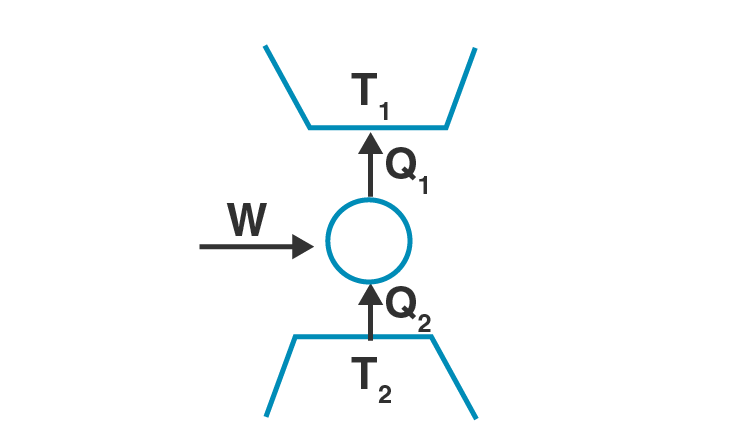If W > 0, then possibilities are:

a) Q1 > Q2 > 0

b) Q2 > Q1 > 0

c) Q2 < Q1 < 0

d) Q1 < 0, Q2 > 0

a) Q1 > Q2 > 0

c) Q2 < Q1 < 0

12.12. Can a system be heated and its temperature remains constant?

For temperature to remain constant, the work done by the system against the surrounding should compensate with the heat that is supplied.

According to the given information,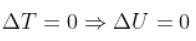Therefore,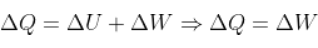12.13. A system goes from P to Q by two different paths in the P-V diagram as shown in the figure. Heat given to the system in the path 1 is 1000 J. The work done by the system along path 1 is more than path 2 by 100 J. What is the heat exchanged by the system in path 2?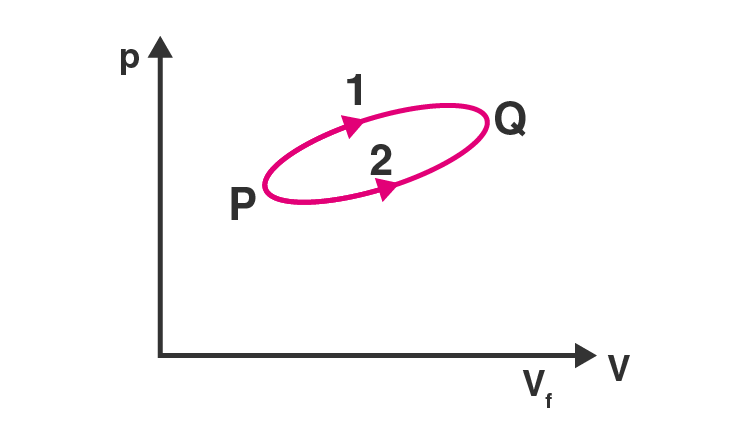From path 1, Q1 = +1000 J

Work done = W1 – W2 = 100

Where,

W1 is the work done through path 1

W2 is the work done through path 2

Therefore, W2 = W1 – 100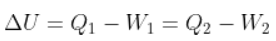Substituting the values, we get Q2 = 900 J

12.14. If a refrigerator’s door is kept open, will the room becomes cool or hot? Explain.

If a refrigerator’s door is kept open, the room becomes hot because the amount of heat absorbed by the refrigerator and the work done by the refrigerator will be rejected to the room.

12.15. Is it possible to increase the temperature of a gas without adding heat to it? Explain.

Yes, it is possible to increase the temperature of a gas without adding heat to it. This is possible during adiabatic compression.

12.16. Air pressure in a car tyre increases during driving. Explain.

Air pressure in a car tyre increases during driving while the volume remains constant which is based on the Charle’s law. Pressure is proportional to the temperature. Therefore, the pressure of the gas increases.

12.17. Consider a Carnot’s cycle operating between T1 = 500K and T2 = 300K producing 1 kJ of mechanical work per cycle. Find the heat transferred to the engine by the reservoirs.

Given,

Temperature of the source, T1 = 500 K

Temperature of the sink, T2 = 300 K

Work done per cycle, W = 1 kJ = 1000 J

Heat transferred to the engine per cycle, Q1 =?

Efficiency of a Carnot engine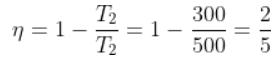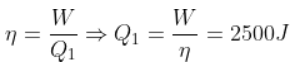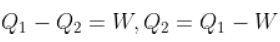= 2500 J – 1000 J = 1500 J

12.18. A person of mass 60 kg wants to lose 5 kg by going up and down a 10 m high stairs. Assume he burns twice as much fat while going up than coming down. If 1 kg of fat is burnt on expending 7000 kilocalories, how many times must he go up and down to reduce his weight by 5 kg?

Given,

Height of the stairs, h = 10 m

Work done to burn 5 kg of fat = (5)(7000 × 103)(4.2) = 147 × 106 J

Work done towards burning of fat in one trip = mgh + 1/2 mgh = 3/2 mgh = (3/2)(60)(10)(10) = 9 × 103 J

Therefore, no.of trips required = 16.3 × 103 times.

12.19. Consider a cycle tyre being filled with air by a pump. Let V be the volume of the tyre and at each stroke of the pump ∆V of air is transferred to the tube adiabatically. What is the work done when the pressure in the tube is increased from P1 to P2?

Following is the equation before and after the stroke: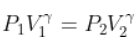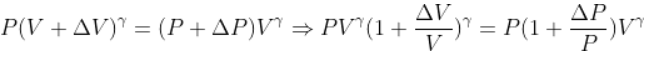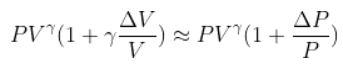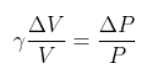Therefore, work done is given as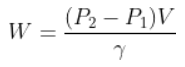12.20. In a refrigerator, one removes heat from a lower temperature and deposits to the surroundings at a higher temperature. In this process, mechanical work has to be done, which is provided by an electric motor. If the motor is of 1kW power, and heat is transferred from -3oC to 27oC, find the heat taken out of the refrigerator per second assuming its efficiency is 50% of a perfect engine.

Efficiency of the Carnot engine is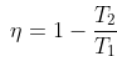Where

T1 = 300 K

T2 = 270 K

It is given that efficiency is 50% = 0.5

Efficiency of refrigerator = 0.05

We know that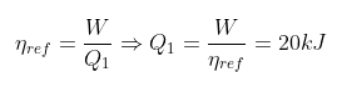Therefore, the efficiency of the refrigerator is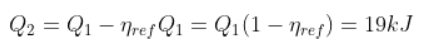12.21. If the coefficient of performance of a refrigerator is 5 and operates at the room temperature, find the temperature inside the refrigerator.

To find the temperature inside the refrigerator, we need to find the work done

W = 0

Q1 = Q2

β = ∞

Where β is the coefficient of performance

Given,

T1 = 27 + 273 = 300 K

Coefficient of performance of refrigerator ω = 5

Coefficient of performance is given as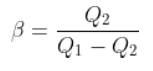Using the above equation, the temperature can be determined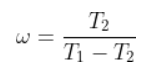T2 = -23oC.

12.22. The initial state of a certain gas is Pi, Vi, Ti. It undergoes expansion till its volume becomes Vf. Consider the following two cases:

a) the expansion takes place at constant temperature

b) the expansion takes place at constant pressure

Plot the P-V diagram for each case. In which of the two cases, is the work done by the gas more?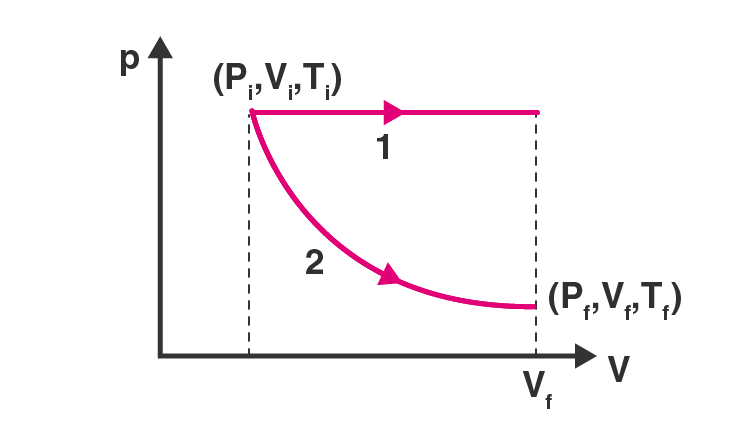12.23. Consider a P-V diagram in which the path followed by one mole of a perfect gas in a cylindrical container is shown in the figure.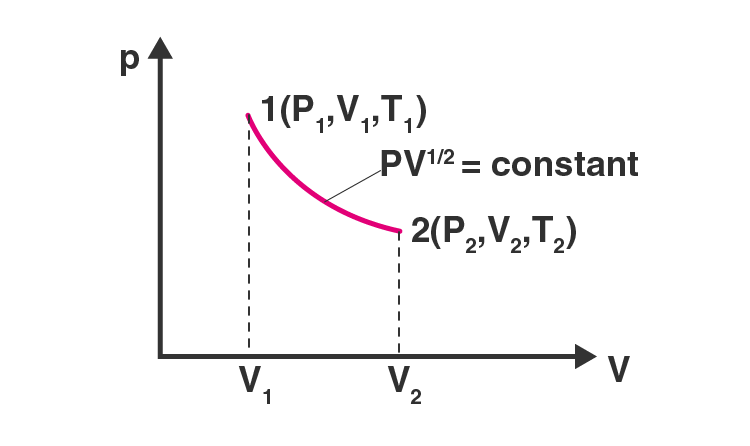a) find the work done when the gas is taken from state 1 to state 2

b) what is the ratio of temperature T1/T2 if V2 = 2V1

c) given the internal energy for one mole of gas at temperature T is (3/2)RT, find the heat supplied to the gas when it is taken from state 1 to 2 with V2 = 2V1.

Given,

PV1/2 = K = constant

a) Work done for the process 1 to 2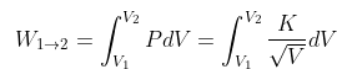Solving the above equation, we get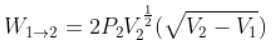b) Ideal gas equation is

PV = nRT

T = PV/nR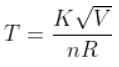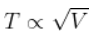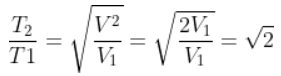c) Internal energy, U = (3/2)RT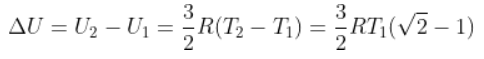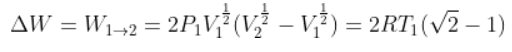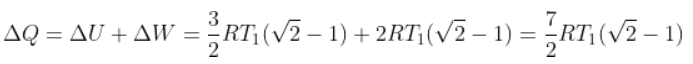12.24. A cycle followed by an engine is shown in the figure.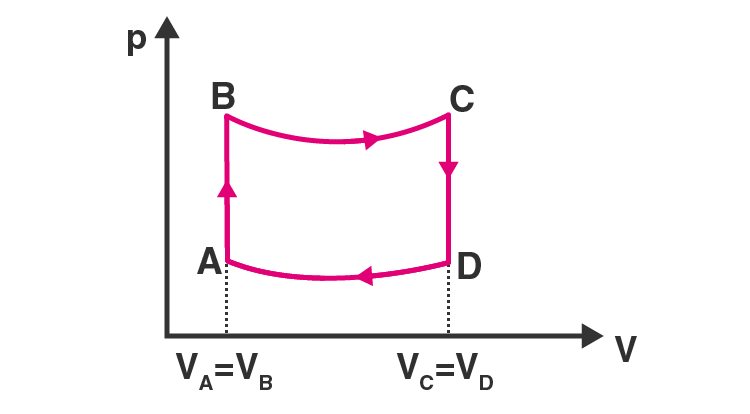A to B: volume constant

C to D: volume constant

VC = VD = 2VA = 2VB

a) in which part of the cycle heat is supplied to the engine from outside?

b) in which part of the cycle heat is being given to the surrounding by the engine?

c) what is the work done by the engine in one cycle in terms of PA, PB, VA?

d) what is the efficiency of the engine?

a) For process AB, the heat is supplied to the engine from outside.

dQ = dU + dW

dQ = dU = change in internal energy

b) For process CD, the volume remains constant and pressure decreases as the temperature for this process decreases.

c) Work  done by the engine in one cycle in terms of PA, PB, VA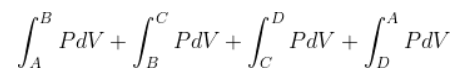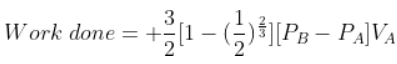d) Efficiency is given as: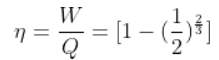12.25. A cycle followed by an engine is shown in the figure. Find heat exchanged by the engine, with the surroundings for each section of the cycle considering Cv = (3/2)R.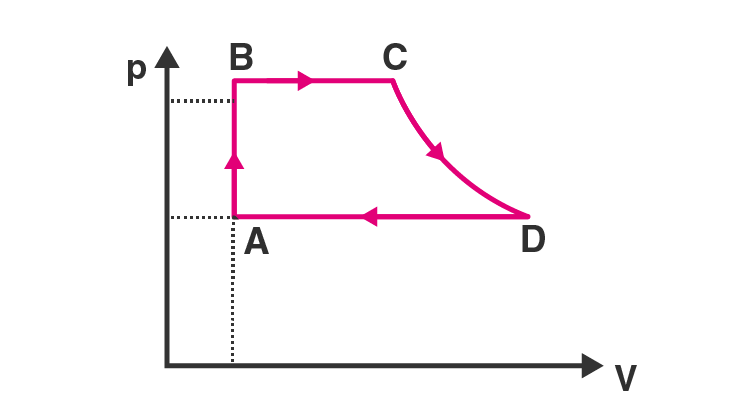AB: constant volume

BC: constant pressure

DA: constant pressure

a) AB: constant volume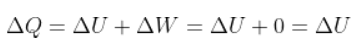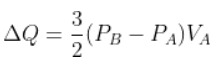b) BC: constant pressure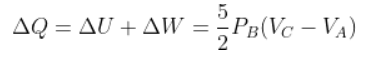c) CD: adiabatic QCD = 0

d) DA: constant pressure, there is compression of the gas from VD to VA at constant pressure PA.

12.26. Consider that an ideal gas is expanding in a process given by P = f(V), which passes through a point (V0, P0). Show that the gas is absorbing heat at (P0, V0) if the slope of the curve P = f(V) is larger than the slope of the adiabat passing through (P0, V0).

Slope of the graph,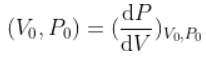Using the above relation, we can find that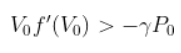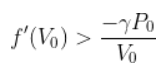12.27. Consider one mole of a perfect gas in a cylinder of unit cross-section with a piston attached. A spring is attached to the piston and to the bottom of the cylinder. Initially the spring is unstretched and the gas is in equilibrium. A certain amount of heat Q is supplied to the gas causing an increase of volume from V0 to V1.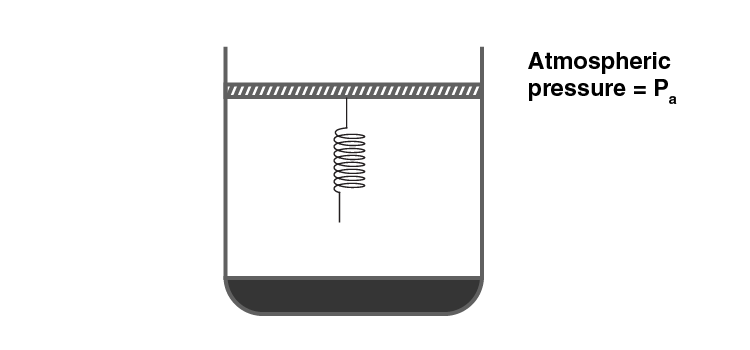a) what is the initial pressure of the system?

b) what is the final pressure of the system?

c) using the first law of thermodynamics, write down the relation between Q, Pa, V, V0, and k.

a) The initial pressure of the system inside the cylinder is = Pa

b) The final pressure of the system is = Pf

Pf = Pa + K (V1 – V0)

Where V1 – V0 is the increase in volume

c) The relation between Q, Pa, V, V0, and k is:

dQ = dU + dW

dU = Cv (T – T0)

Where,

T is the final temperature of the gas

T0 is the initial temperature of the gas

n = 1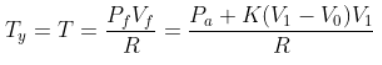Work done by the gas is given as: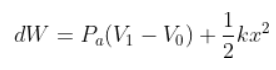dQ = dU + dW

Using this equation, we get the following required relation: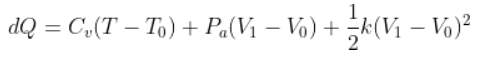### Why Opt for BYJU’S

BYJU’S is India’s best online education provider with innovative approaches to help students learn the concepts thoroughly and thereby increase their grades. To avail of all the study materials, video and animation lessons and exercises provided by us, and for a great learning experience, visit BYJU’S website or download BYJU’S – The Learning App.